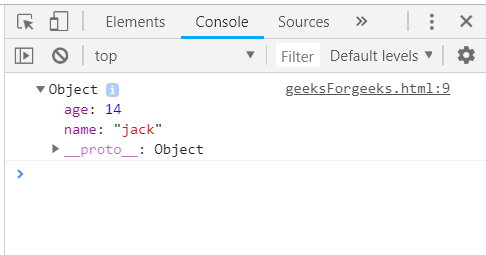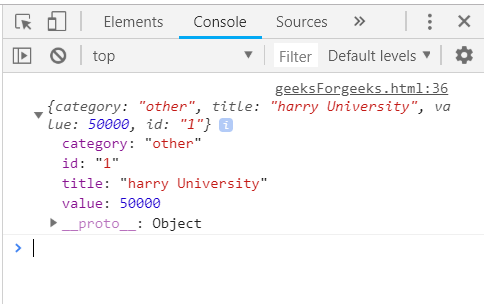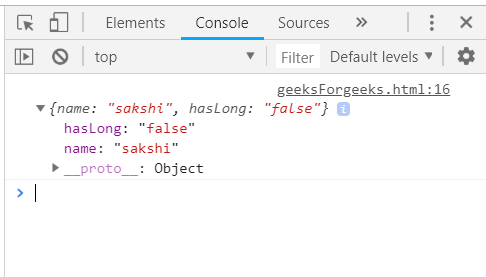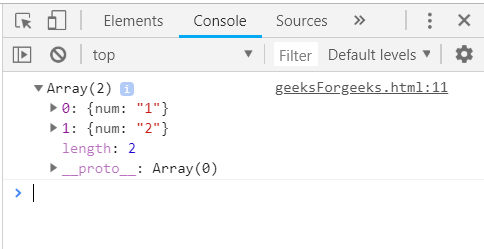# Underscore.js | first() with Examples

The Underscore.js is a JavaScript library that provides a lot of useful functions that helps in the programming in a big way like the map, filter, invoke etc even without using any built-in objects.
The _.first() function is used to return the first element of the array, i.e. the number at the zeroth index. It returns first n elements in the array of m size (n < m) by just passing the variable n in the array. It is a very easy to use function of the underscore.js library and is used widely when working with array elements.

Syntax:

`_.first(array, [n]) `

Parameters: This function accepts two parameter as mentioned above and described below:

• array: This parameter is used to hold the array of elements.
• variable: It tells the number of elements wanted.

Return values: This function returns the array of elements.

Passing an array to the _.first function(): The ._first() function will return the first element along with all it’s properties of the array passed. Like here, the elements has 2 properties, the name and the age so, the final result will contain both of these properties of the first element as the variable n is not passed here.

Example:

 `<``html``> ` `    ``<``head``> ` `        ``<``script` `type``=``"text/javascript"` `src``= ` `        ``"https://cdnjs.cloudflare.com/ajax/libs/underscore.js/1.9.1/underscore.js"``> ` `    `` ` `    ``<``body``> ` `        ``<``script` `type``=``"text/javascript"``> ` `        ``console.log(_.first([{name: 'jack', age: 14},  ` `                             ``{name: 'jill', age: 15},  ` `                             ``{name: 'humpty', age: 16}])); ` `        `` ` `    `` ` `                     `

Output:Passing a structure to _.first() function: The ._first() function will return the first element along with all it’s properties of the array passed as the variable n is not passed here. Like here, each elements has four properties, the category, the title, the value and the id. So, the final result will contain all of these properties of the first element.

Example:

 `<``html``> ` `    ``<``head``> ` `        ``<``script` `type``=``"text/javascript"` `src` `=  ` `        ``"https://cdnjs.cloudflare.com/ajax/libs/underscore.js/1.9.1/underscore-min.js"` `> ` `        `` ` `    `` ` `    ``<``body``> ` `        ``<``script` `type``=``"text/javascript"``> ` `            ``var goal  = [ ` `            ``{ ` `                ``"category" : "other", ` `                ``"title" : "harry University", ` `                ``"value" : 50000, ` `                ``"id":"1" ` `            ``}, ` `            ``{ ` `                ``"category" : "traveling", ` `                ``"title" : "tommy University", ` `                ``"value" : 50000, ` `                ``"id":"2" ` `            ``}, ` `            ``{ ` `                ``"category" : "education", ` `                ``"title" : "jerry University", ` `                ``"value" : 50000, ` `                ``"id":"3" ` `            ``}, ` `            ``{     ` `                ``"category" : "business", ` `                ``"title" : "Charlie University", ` `                ``"value" : 50000, ` `                ``"id":"4" ` `            ``} ` `        ``] ` `        ``console.log(_.first(goal)); ` `        `` ` `    `` ` ` `

Output:Passing an array with one property as true/false to the _.every() function: This will work exactly the same as the above two examples. The false/true property will only be displayed of the first element. Here, this property will not be logically used.

Example:

 `<``html``> ` `    ``<``head``> ` `        ``<``script` `type``=``"text/javascript"` `src` `=  ` `        ``"https://cdnjs.cloudflare.com/ajax/libs/underscore.js/1.9.1/underscore-min.js"` `> ` `        `` ` `    `` ` `    ``<``body``> ` `        ``<``script` `type``=``"text/javascript"``> ` `            ``var people = [ ` `            ``{"name": "sakshi", "hasLong": "false"}, ` `            ``{"name": "aishwarya", "hasLong": "true"}, ` `            ``{"name": "akansha", "hasLong": "true"}, ` `            ``{"name": "preeti", "hasLong": "true"} ` `        ``] ` `         ``console.log(_.first(people)); ` `        `` ` `    `` ` ` `

Output:Passing an array with the variable n to the _.first() function: To have more than one element which is the first element, just pass the number and get result. Remember that the elements will always come from the start of the array.

Example:

 `<``html``> ` `    ``<``head``> ` `        ``<``script` `type``=``"text/javascript"` `src` `=  ` `        ``"https://cdnjs.cloudflare.com/ajax/libs/underscore.js/1.9.1/underscore-min.js"` `> ` `        `` ` `    `` ` `    ``<``body``> ` `        ``<``script` `type``=``"text/javascript"``> ` `             ``var users = [{"num":"1"}, {"num":"2"},  ` `             ``{"num":"3"}, {"num":"4"}, {"num":"5"}]; ` `        ``console.log(_.first(users, 2)); ` `        `` ` `    `` ` ` `

Output:My Personal Notes arrow_drop_upCheck out this Author's contributed articles.

If you like GeeksforGeeks and would like to contribute, you can also write an article using contribute.geeksforgeeks.org or mail your article to contribute@geeksforgeeks.org. See your article appearing on the GeeksforGeeks main page and help other Geeks.

Please Improve this article if you find anything incorrect by clicking on the "Improve Article" button below.

Improved By : shubham_singh

Article Tags :

Be the First to upvote.

Please write to us at contribute@geeksforgeeks.org to report any issue with the above content.